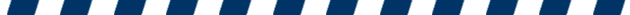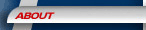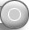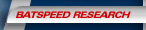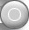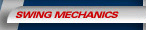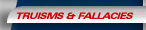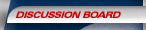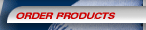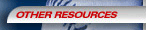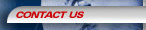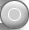Re: Re: Re: Re: Re: Re: pitch angle/gravity etc.

Posted by: () on Wed Jan 23 12:46:50 2002

Could someone help me out with the math or with the amount of drop caused by gravity during the period of time a pitch is traveling to the plate?
> > > > > >
> > > > > > Thanks,
> > > > > > Mark H.
> > > > >
> > > > > OK, found that. Now, anyone have anything to convince a fastpitch coach that, even in fastpitch, most, if not all, pitches have a downward angle as they cross the plate? I've done the math but I would like some "outside" expert testimony to bolster my contention.
> > > > >
> > > > > Thanks,
> > > > > Mark H.
> > > >
> > > > Mark -
> > > > I don't have any outside experts to refer you to. Sorry. But in a discussion on FastPitchForum a while back, it was made clear that it is possible to throw a softball with an upward trajectory at homeplate at the high end of pitching velocities (60+MPH). I'm not talking 'riseball' due to spin, just a fastball released slightly upward. I suspect just about everything else would have some downward component, but maybe not as much as in baseball where the release point is higher to start with. Baseball is more 'forward and down', fastpitch is more 'up then down'.
> > >
> > >
> > > Major Dan,
> > >
> > > This is what I came up with. Critiques are welcome from all.
> > >
> > > Given a fastpitch pitcher,s stride and release point, the ball must travel about 39 feet to reach the plate given a college 43 foot rubber. Balls are generally struck a little in front of the plate, so we will assume a flight distance of 38 feet on average from release to contact area. At 60 mph the pitch is traveling 88 feet per second. We will use 60 since a slower speed will only increase the down angle as the pitch crosses the hitting area. At 88 feet per second the pitch will take .43 seconds from release to contact.
> > >
> > > Where d is distance in meters, 0.5 is the constant, .43 is t or time, and 9.8 is g or gravity (closer than 10)
> > > Therefore the forumla looks like this: d= 0.5*9.8*.43 squared
> > > d=0.5*9.8*.1849
> > > d=.90601 meters or 2.97 feet of drop due to gravity in an 60mph college pitcher
> > >
> > > The drop would of course be less at the 40' pitching distance than the 43' distance. All of this means, in the absence of spin induced forces, that a pitch must be thrown on an initial trajectory aimed 2.97 feet higher than it's intended target. Thus, even a pitch at the shoulders will be leveling off and perhaps just starting to come down as it crosses the plate given a release point near the knee. A pitch at the knees (slightly below release point) must go down longer than it goes up since the release point is above the target, so for over half it's flight, it is headed down. Downspin, inducing extra down force, would have the effect of increasing the steepness of both the initial up trajectory and the final down angle as the pitch passes over the plate. A slower speed would increase t and cause the same thing-a steeper down angle through the hitting zone or over the plate. Is this clear?
> > >
> > > The following is pulled from a website:
> > >
> > > The distance which a free-falling object has fallen from a position of rest is also dependent upon the time of fall. This distance can be computed by use of a formula; the distance fallen after a time of t seconds is given by the formula.
> > >
> > > d = 0.5 * g * t2
> > >
> > > where g is the acceleration of gravity (approximately 10 m/s/s on Earth). Example calculations for the distance fallen by a free-falling object after one and two seconds are shown below.
> > >
> > >
> > > Example Calculations:
> > > At t = 1 s
> > > d = (0.5) * (10 m/s2) * (1 s)2 = 5 m
> > >
> > >
> > >
> > > At t = 2 s
> > >
> > > d = (0.5) * (10 m/s2) * (2 s)2 = 20 m
> > >
> > >
> > >
> > > At t = 5 s
> > >
> > > d = (0.5) * (10 m/s2) * (5 s)2 = 125 m
> > >
> > >
> > >
> > The drop from rest times will be the same as the drop from a pitch thrown dead level with the ground. Gravity is a vector force that only works on the vertical component. Any sideways velocity does not figure in.
> > If a fp pitcher throws with an upward angle, that angle will be countered by gravity until gravity wins. At that point it will arc over and start to accelerate downward according to your formula.
> > The question is how do you calculate the upward force of the pitch - 60 mph total velocity is maybe 50 mph horizontal and 10 mph vertical (the division is pure guesswork for conjecture). Gravity must neutralize the upward force (decellerate the ball) before the ball starts accelerating. I'm not sure if you can do the simple thing- calculate how much rise there would be without gravity (angle of ascent) then subtract the drop due to gravity to get a net drop (rise minus gravity drop). I think that would work but I don't have time now to check my physics.
>
>
> This is a simple free-fall physics problem:
>
> d=vavg*t
> =(0.5*(vi+vf))*t
> =(0.5vi+0.5vf)*t
> =(vi-0.5vi+0.5vf)*t
> =(vi+0.5*(vf-vi))*t
> =(vi+0.5*(vf-vi)/t*t*t
> =(vi+0.5*a*t)*t
> =vi*t + 0.5at^2
>
> vi is initial vertical velocity
> a is vertical acceleration = -9.81m/s^2
> t is flight time = 0.43s
>
> Therefore, d=vi*0.43 + 0.5*(-9.81)*0.43^2
> =0.43vi - 0.91m
>
> Now, for the ball to rise, d=0.43vi-0.91m>0
> 0.43vi>0.91m
> vi>2.1m/s
>
> So, with a flight time of 0.43s, the ball would have to be released with an initial upward velocity of at least 2.1m/s to have the ball contact the bat at, or above, the vertical release point of the pitcher.
>
> Similarly,
> The pitchers vertical release velocity, such that the ball is always rising to the plate is governed by the condition vf=0:
>
> vf=vi+at
> 0=vi+(-9.81m/s)*0.43s
> vi=4.22m/s
>
> So, for a flight time of 0.43s, if the ball is thrown with at least 4.22m/s initial velocity upwards, then the ball will rise continuously to the plate.

Thanks for your help Mike. Considering a release point between the knee and the hip, an initial speed of 60 mph and the 4.22m/s initial velocity upward you mentioned, where would that pitch cross the plate relative to the strike zone?

Thanks,
Mark H.

Followups:

Post a followup:
Name:
E-mail:
Subject:
Text:

Anti-Spambot Question:This song is traditionally sung during the 7th inning stretch?    All My Roudy Friends    Take Me Out to the Ballgame    I Wish I was in Dixie    Hail to the Chief

[   SiteMap   ]